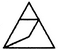# Non Verbal Reasoning - Shape Construction

### Exercise :: Shape Construction - Section 2

In each of the following questions, a set of five alternative figures 1, 2, 3, 4 and 5 followed by a set of four alternatives (A), (B), (C) and (D) is provided. It is required to select the alternative which represents three out of the five alternative figures which when fitted into each other would form a equilateral triangle.

1.

Select the alternative which represents three out of the five alternative figures which when fitted into each other would form a equilateral triangle.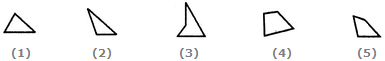A. 234 B. 134 C. 345 D. 245

Explanation: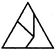2.

Select the alternative which represents three out of the five alternative figures which when fitted into each other would form a equilateral triangle.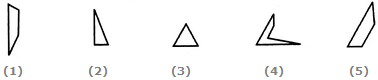A. 124 B. 135 C. 134 D. 345

Explanation: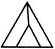3.

Select the alternative which represents three out of the five alternative figures which when fitted into each other would form a equilateral triangle.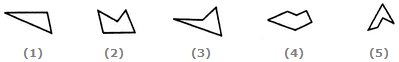A. 135 B. 124 C. 245 D. 345

Explanation: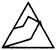4.

Select the alternative which represents three out of the five alternative figures which when fitted into each other would form a equilateral triangle.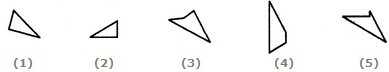A. 125 B. 235 C. 145 D. 245

Explanation: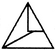5.

Select the alternative which represents three out of the five alternative figures which when fitted into each other would form a equilateral triangle.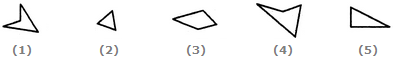A. 124 B. 234 C. 245 D. 345